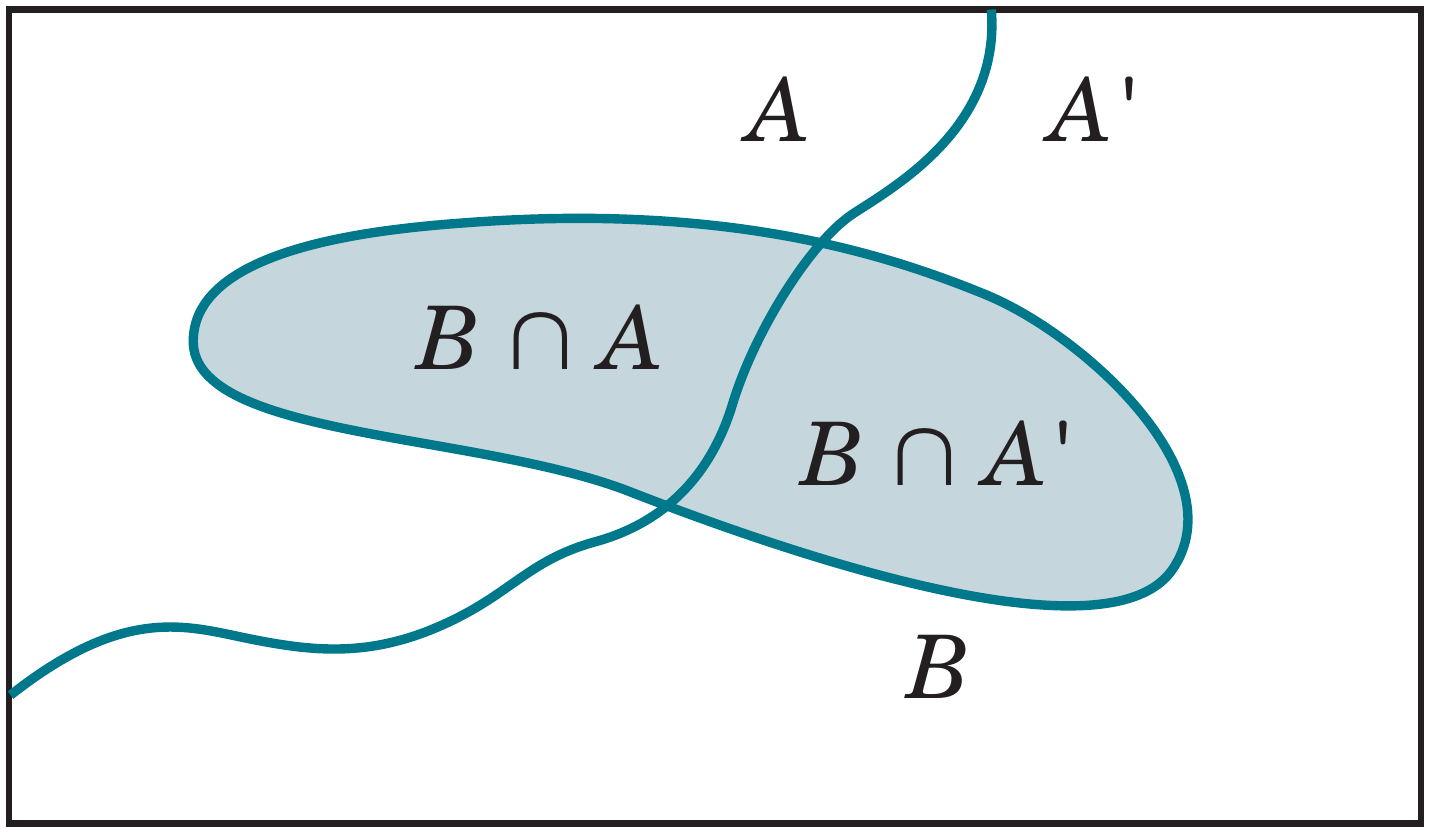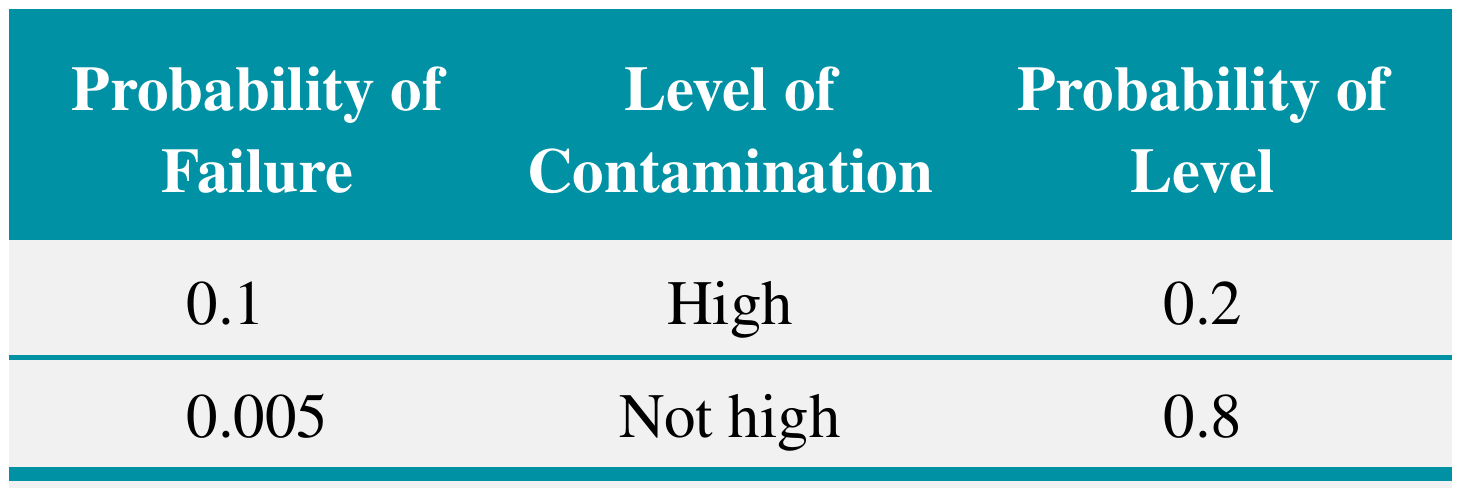# Total Probability, Independence and Bayes' theorem

02/08/2021

## Instructions:

Use the left and right arrow keys to navigate the presentation forward and backward respectively. You can also use the arrows at the bottom right of the screen to navigate with a mouse.

FAIR USE ACT DISCLAIMER:
This site is for educational purposes only. This website may contain copyrighted material, the use of which has not been specifically authorized by the copyright holders. The material is made available on this website as a way to advance teaching, and copyright-protected materials are used to the extent necessary to make this class function in a distance learning environment. The Fair Use Copyright Disclaimer is under section 107 of the Copyright Act of 1976, allowance is made for “fair use” for purposes such as criticism, comment, news reporting, teaching, scholarship, education and research.

## Outline

• The following topics will be covered in this lecture:

• Multiplication Rule
• Total Probability Rule
• Independence
• Bayes' theorem

## Intersections of Events and Multiplication Rule

• Recall that last time we discussed the probability of the intersection of two events.
• Let us suppose that $$A$$ and $$B$$ are events for which $$P(A)\neq 0$$ and $$P(B)\neq 0$$.
• Using the definition of conditional probability $P(B|A)=\frac{P(A\cap B)}{P(A)}$
• We can solve for the intersection of events $P(A \cap B) = P(B\vert A) P(A)$
• Similarly, from the same definition of conditional probability we have $P(A|B)=\frac{P(A\cap B)}{P(B)}$
• which implies that $P(A \cap B) = P(A\vert B) P(B)$
• We can provide a formula known as the multiplication rule
Probability of an Intersection: $P(A \cap B) = P(B\vert A) P(A) = P(A\vert B) P(B)$

## Intersections of Events – example

• EXAMPLE: The probability that the first stage of a numerically controlled machining operation for high-rpm pistons meets specifications is 0.90.

• Failures are due to metal variations, fixture alignment, cutting blade condition, vibration, and ambient environmental conditions.

• Given that the first stage meets specifications, the probability that a second stage of machining meets specifications is 0.95.

• Question: Using the multiplication rule,

$P(A \cap B) = P(B\vert A) P(A) = P(A\vert B) P(B)$ what is the probability that both stages meet specifications?

• Let the events be $$A=$$"first stage meets specifications" and $$B=$$"second stage meets specifications".
• The probability requested is $$P(A \text{ and }B)$$
• where $$P(A)=0.90$$
• and $$P(B|A)=0.95$$
• Using the multiplication rule, we get \begin{align} P(A \cap B) &= P(B\vert A) P(A) \\ &= 0.95(0.90) = 0.855 \end{align}
• Note: although it is also true that $$P(A \cap B) = P(A | B)P(B)$$, the information provided in the problem does not match this second formulation.

## Total Probability Rule

• So far we have described probabilities of events in terms of the probabilities of union or intersections of events, i.e.:
• Addition rule: $$P(A\cup B) = P(A) + P(B) - P(A\cap B)$$;
• Conditional probability: $$P(A\vert B) = \frac{P(A\cap B)}{P(B)}$$; and the
• Multiplication rule: $$P(A\cap B) = P(A\vert B)P(B)$$.
• What if we want to recover the probability of single event $$P(B)$$ given several conditions?Courtesy of Montgomery & Runger, Applied Statistics and Probability for Engineers, 7th edition

• For any event $$B$$, we can write $$B$$ as the union of the part of $$B$$ in $$A$$ and the part of $$B$$ in $$A′$$. That is $B=(A\cap B)\cup(A'\cap B)$
• Because $$A$$ and $$A′$$ are mutually exclusive
• $$A \cap B$$ and $$A' \cap B$$ are mutually exclusive
• So we can use the addition rule for mutually exclusive events as \begin{align} P(B)&=P((A\cap B)\cup(A'\cap B))\\ &=P(A\cap B)+P(A'\cap B)\end{align}
• Using the multiplication rule on each term of $$P(B)$$ we get $P(B)=P(B|A)P(A)+P(B|A')P(A')$
• Total Probability Rule (Two Events) For any two events $$A$$ and $$B$$
• \begin{align} P(B)=P(B\cap A)+P(B\cap A')=P(B|A)P(A)+P(B|A')P(A') \end{align}

## Total Probability Rule – exampleCourtesy of Montgomery & Runger, Applied Statistics and Probability for Engineers, 7th edition

• Suppose that in semiconductor manufacturing, the probability is 0.10 that a chip subjected to high levels of contamination during manufacturing has a product failure.
• The probability is 0.005 that a chip not subjected to high contamination levels during manufacturing has a product failure.
• In a particular production run, 20% of the chips are subject to high levels of contamination.
• Question: using the total probability rule \begin{align} P(B)=P(B\cap A)+P(B\cap A')=P(B|A)P(A)+P(B|A')P(A'), \end{align}
• what is the total probability of a chip failure?
• Lets denote the events be $$F=$$"product fails" and $$H=$$"chip is exposed to high levels of contamination".
• From the table we can extract some pieces of information
• $$P(H)=0.2$$ and $$P(H')=0.8$$
• $$P(F|H)=0.10$$ and $$P(F|H')=0.005$$
• We can use the total probability rule on $$P(F)$$ in terms of conditional probabilities $P(F)=P(F|H)P(H)+P(F|H')P(H')$
• Then the probability that a product fails is $P(F)=0.10(0.20)+0.005(0.80)=0.024$